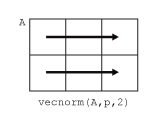# vecnorm

Vector-wise norm

## Syntax

``N = vecnorm(A)``
``N = vecnorm(A,p)``
``N = vecnorm(A,p,dim)``

## Description

example

````N = vecnorm(A)` returns the 2-norm or Euclidean norm of `A`:If `A` is a vector, then `vecnorm` returns the norm of the vector.If `A` is a matrix, then `vecnorm` returns the norm of each column.If `A` is a multidimensional array, then `vecnorm` returns the norm along the first array dimension whose size does not equal 1.```

example

````N = vecnorm(A,p)` calculates the generalized vector p-norm.```

example

````N = vecnorm(A,p,dim)` operates along dimension `dim`. The size of this dimension reduces to 1 while the sizes of all other dimensions remain the same.```

## Examples

collapse all

Calculate the 2-norm of a vector corresponding to the point (2,2,2) in 3-D space. The 2-norm is equal to the Euclidean length of the vector, $\sqrt{12}$.

```x = [2 2 2]; n = vecnorm(x)```
```n = 3.4641 ```

Calculate the 1-norm of the vector, which is the sum of the element magnitudes.

`n = vecnorm(x,1)`
```n = 6 ```

Calculate the 2-norm of the columns of a matrix.

`A = [2 0 1;-1 1 0;-3 3 0]`
```A = 3×3 2 0 1 -1 1 0 -3 3 0 ```
`n = vecnorm(A)`
```n = 1×3 3.7417 3.1623 1.0000 ```

As an alternative, you can use the `norm` function to calculate the 2-norm of the entire matrix.

## Input Arguments

collapse all

Input array, specified as a vector, matrix, or multidimensional array. By convention, `vecnorm` returns `NaN` values if the vector being operated on contains a `NaN` value.

Data Types: `single` | `double`
Complex Number Support: Yes

Norm type, specified as `2` (default), a positive scalar, or `Inf`.

Dimension to operate along, specified as a positive integer scalar. If you do not specify a value, then the default is the first array dimension whose size does not equal 1.

Dimension `dim` indicates the dimension whose length reduces to 1. In other words, `size(N,dim)` is `1`, while the sizes of all other dimensions remain the same.

Consider a two-dimensional input array, `A`:

• `vecnorm(A,p,1)` calculates the norm of each column.• `vecnorm(A,p,2)` calculates the norm of each row.`vecnorm` returns `abs(A)` when `dim` is greater than `ndims(A)` or when `size(A,dim)` is `1`.

Data Types: `single` | `double` | `int8` | `int16` | `int32` | `int64` | `uint8` | `uint16` | `uint32` | `uint64`

collapse all

### Euclidean Norm

The Euclidean norm (also called the vector magnitude, Euclidean length, or 2-norm) of a vector `v` with `N` elements is defined by

`$‖v‖=\sqrt{\sum _{k=1}^{N}{|{v}_{k}|}^{2}}\text{\hspace{0.17em}}.$`

### General Vector Norm

The general definition for the p-norm of a vector `v` that has `N` elements is

`${‖v‖}_{p}={\left[\sum _{k=1}^{N}{|{v}_{k}|}^{p}\right]}^{\text{\hspace{0.17em}}1/p}\text{\hspace{0.17em}},$`

where `p` is any positive real value or `Inf`. Some interesting values of `p` are:

• If `p = 1`, then the resulting 1-norm is the sum of the absolute values of the vector elements.

• If `p = 2`, then the resulting 2-norm gives the vector magnitude or Euclidean length of the vector.

• If `p = Inf`, then ${‖v‖}_{\infty }={\mathrm{max}}_{i}\left(|v\left(i\right)|\right)$.

## Version History

Introduced in R2017b